Browse by Topic
Related Topics

# Jacob Bernoulli’s Legacy in Mathematica

January 16, 2015, marks the 360th birthday anniversary of Jacob Bernoulli (also James, or Jacques).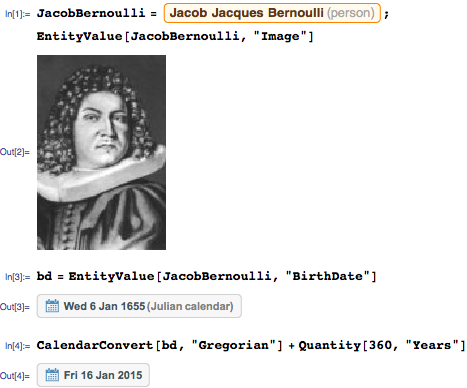Jacob Bernoulli was the first mathematician in the Bernoulli family, which produced many notable mathematicians of the seventeenth and eighteenth centuries.

Jacob Bernoulli’s mathematical legacy is rich. He introduced Bernoulli numbers, solved the Bernoulli differential equation, studied the Bernoulli trials process, proved the Bernoulli inequality, discovered the number e, and demonstrated the weak law of large numbers (Bernoulli’s theorem).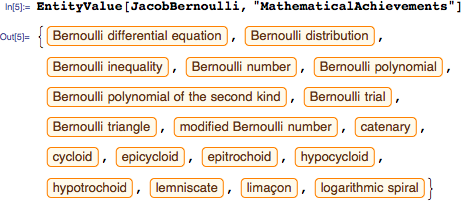Bernoulli’s treatise Ars Conjectandi (i.e. The Art of Conjecturing) was posthumously published in 1713, eight years after his demise, and was written in Latin, science’s lingua franca of the time. It is considered a seminal work of mathematical probability. Its importance is witnessed, in part, by its translations to French by G. Le Roy in 1801, and, recently, to English by E. D. Sylla in 2005.

The Art of Conjecturing comprises four parts. The first part reproduces Christiaan Huygens’ De Ratiociniis in Ludo Aleae (On Reasoning in Games of Chance), with extensive commentary from Bernoulli and detailed solutions of Huygens’ five problems, posed at the end of Huygens’ work with answers, but without derivations. In the first part, Bernoulli also derives the probability that at least m successes will occur in n independent trials with success probability of p: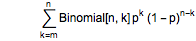The second part, “The Doctrine of Permutations and Combinations,” is devoted to combinatorics and to the study of figurate numbers, i.e. numbers that can be represented by a regular geometrical arrangement of equally spaced points: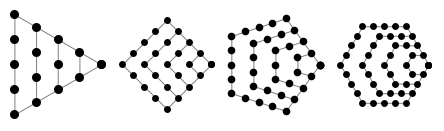It is here that Bernoulli introduces Bernoulli numbers. He starts by noting the identity among binomial coefficients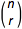, namely that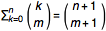.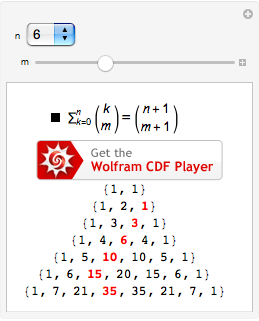Bernoulli knew that for a fixed m, binomial coefficient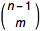is a polynomial in n, namely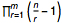. This identity allows him to solve for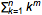. He gives a table of results for 0≤m≤10.

To reproduce Bernoulli’s table, define a function to construct equations for the sum of powers: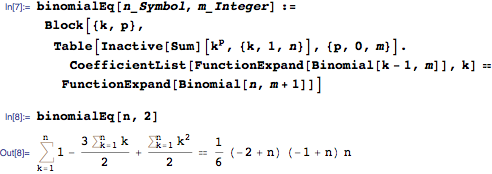Solving for the sum of powers: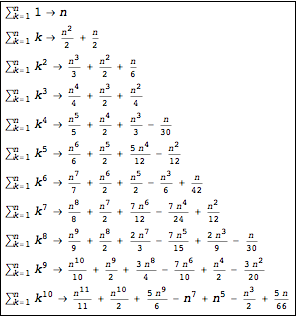Bernoulli writes, “[W]hoever has examined attentively the law of the progression herein can continue the Table further without these digressions for computations” by making the following educated guess: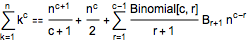He notes that coefficients Br+1 do not depend on n, and can be computed recursively by substituting n==1 into the above equation.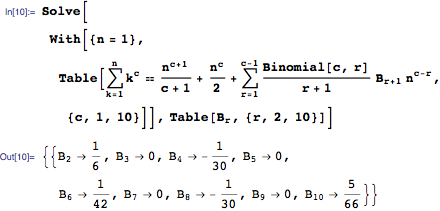These coefficients are the celebrated Bernoulli numbers, which have found their way into many areas of mathematics [e.g. see mathoverflow.net].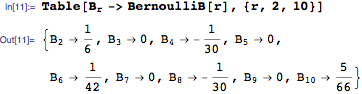In the second part of his book, Bernoulli counts the number of permutations, the number of permutations in sets with repetitions, the number of choosing objects from a set, etc., which he later applies to compute probabilities as the ratio of the number of configurations of interest to the total number of configurations.

In part three, Bernoulli applies results from the first two chapters to solve 24 problems related to games of chance. A recurring theme in these problems is a sequence of independent 0 or 1 outcomes, which bears the name of Bernoulli trial, or Bernoulli process. I thought Jacob Bernoulli’s birthday anniversary to be an apt occasion to explore his problems with Mathematica.

For example, problem 9 asks to find the expected payout in a three-player game. Players alternately draw cards without replacement from a pack of twenty cards, of which ten are face cards. When the cards are exhausted, winnings are divided among all those who hold the highest number of face cards.

With c1, c2, and c3 denoting the number of face cards each player has, the payout of the first player is: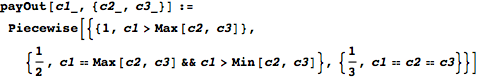After the pack of twenty has been distributed, the first and the second players each receive seven cards, but the third one only receives six. The tally vector of face cards received by each player follows MultivariateHypergeometricDistribution: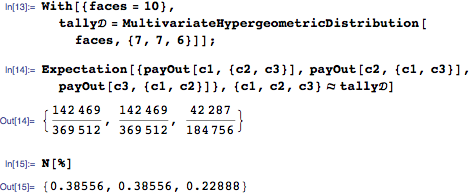This and other problems are stated and solved in the accompanying Mathematica notebook.

The concluding part four of Ars Conjectandi discusses uses of probability in civil, moral, and economic matters. Here Bernoulli argues that the probability reflects our incomplete knowledge of the state of the world, and unlike in a game of chance, where probabilities can be determined by finding the proportion that configurations of interest take in the whole set of possible configurations, the probabilities here cannot be a priori established. Bernoulli argues that these unknown probabilities can be inferred from past outcomes.

He proves the weak law of large numbers, asserting that the observed frequency of successes in n independent trials where the probability of success equals p will converge to p as the number of trials grows. Thus, you can estimate p arbitrarily accurately by running a sufficient number of trials. Specifically, for any δ and ε, there exists a large enough sample size n that: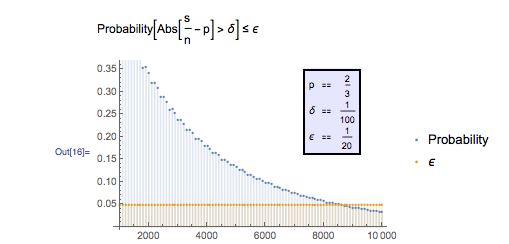The demonstration “Simulated Coin Tossing Experiments and the Law of Large Numbers” by Ian McLeod, among others, explores this convergence.